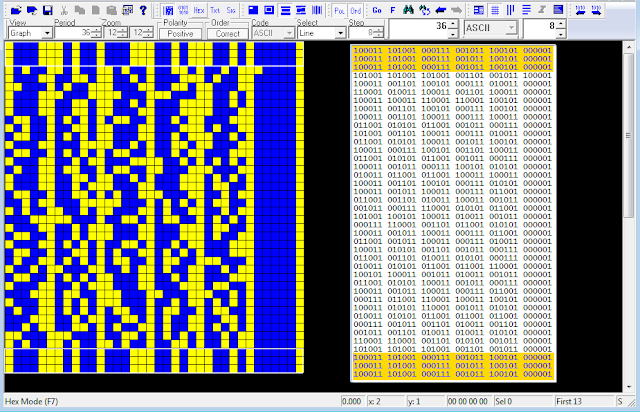## 21 October 2021

### CIS MPSK-16, a POSTNET 2-of-5 variant?

As for the 6-bit encoding used in CIS MPSK-16 streams, see this post for background, my friend Nicholas suggested that most likely it's a variant of the 2-out-of-5 code , a constant-weight code that provides ten possible combinations of two bits and is used for representing the decimal digits using five bits. A such coding works as follows: each bit position is assigned a numerical weight, i.e. a value, e.g. seen from the left '01236', so if bit 4 (the leftmost bit) is a '1' and bit 2 is also a '1' then the combination '10100' would yield 0+2 = 2.

The codeword format is XXXXXS, where X = '0' or '1' and S is the stop bit, always '1', so in order to decode it you will have to invert the polarity of the bitstream 1 to obtain a 1:0 ratio of 2:3, indeed, I found a ratio 1:0 = 3:2 in reverse polarity.Fig. 1

Using the table shown in the cited post, after  invert the polarity and remove the stop bits, I tried to apply the  POSTNET 74210 code  to the source message (assuming 11111/00000 is the codeword used for the separator character):Fig. 2 - 2-out-of-5 code

00111 = A     11000 = 0 (---)
01011 = B     10100 = 9 (7+2)
01101 = C     10010 = 8 (7+1)
01110 = D     10001 = 7 (7+0)
10011 = E     01100 = 6 (4+2)
10101 = F     01010 = 5 (4+1)
10110 = G     01001 = 4 (4+0)
11001 = H     00110 = 3 (2+1)
11010 = I     00101 = 2 (2+0)
11100 = L     00011 = 1 (1+0)

As you see, applying the POSTNET 74210 coding to the source message, from the fourth group of the first line (and form the first group of the last line) yields the 5-digit group 99932  where 32 is just the total number of the groups within the message:

79128 79128 79128 99932
73814 04737 77008 73818
73717 65621 93714 65728
71837 65621 72185 46677
73815 72716 63472 21056
98742 10365 72716 62714
75321 63451 45660 87242
65721 72716 16078 75025
45666 12343 23445 00352
99932 79128 79128 79128

However, it remains to be seen whether '999' is actually '000' or "---" (as said, the coding could be a variant of 74210) and the meaning of the other 3 groups (79128) of the first and last line: probably something related to the message itself, perhaps the number of the message?